# Sound radiation of simply supported rectangular plate using finite element method

## Akshaj Kulshreshtha1, Shivam Yadav2, Baij Nath Singh3, Vinayak Ranjan4

1, 2Bennett University, Greater Noida, India

1Corresponding author

Vibroengineering PROCEDIA, Vol. 29, 2019, p. 195-200. https://doi.org/10.21595/vp.2019.21104
Received 20 October 2019; accepted 29 October 2019; published 28 November 2019

43rd International Conference on Vibroengineering in Greater Noida (Delhi), India, November 28-30, 2019

Copyright © 2019 Akshaj Kulshreshtha, et al. This is an open access article distributed under the Creative Commons Attribution License, which permits unrestricted use, distribution, and reproduction in any medium, provided the original work is properly cited.
Views 684
Abstract.

In this paper sound radiation of simply supported rectangular plate has been reported. The novelty in this research paper is a new method is prescribed for sound radiation calculation. The phenomenon of uncoupled structural acoustics have been followed such that structure is the critical part in structural vibration using modal analysis and harmonic response calculation for frequency range of interest 0 to 200 Hz, but in harmonic acoustic analysis the structure does not takes part in physics point of view but is used for only for transferring the normal velocity from wetted surface of the structure to the interface acoustic medium i.e. air. Sound Radiation parameters like Sound Pressure Level, Sound Power Level and Sound Radiation efficiency has been reported. Upon comparing the results with that from I. Zaman’s literature, it is found that the results are quite varying due to the fact the speed of sound in air as taken in literature is unjustified. So true simulation using correct data has been carried out in this paper and sound radiation results has been reported for frequency range of interest 0 to 200 Hz. It is found that the maximum sound pressure level is 72 dB and maximum sound power level is 78 dB which is suitable for noiseless environment.

Keywords: acoustic radiation, sound pressure level, acoustic power, sound power level, radiation efficiency.

#### 1. Introduction

Thin plates are widely used in many industries such as aircraft, automobile due to light weight. which is the cause of vibration and noise. These plate structures are subjected to externally induced vibrations which cause sound radiation. Therefore, the estimation of sound radiation parameters such as Sound pressure level, Sound power level and radiation efficiency are important which are carried in this paper.

The sound radiation of clamped circular plate using Rayleigh surface integral and convolution on pressure impulse response dependent upon the acceleration of vibrating mode shape was carried by Akay et al. . The use of infinitely extended plates for sound radiation calculation was prescribed by Cummings et al. . The sound radiation formula for baffled rectangular plate was prescribed by C. E Wallace et al.  for which ratio between the wavenumbers of acoustic to structure is below unity. The quite simpler formula for sound pressure level for far field acoustic radiation was prescribed by Fahy et al.  which is dependent upon the factor $ka$ where $k$ stands for acoustic wavenumber and $a$ stands for radius of piston. The double layer integral representation of acoustic pressure from simply supported the current paper pertains to far field acoustic radiation of vibrating simply supported rectangular plate placed in unbaffled vibrating plate was presented by Laulagnet et al. . The steps involved in acoustic radiation of 2 dimensional piston by using the Laplace equation as prescribed by Kirkup et al.  made a basis for acoustic radiation of three dimensional structures.

#### 2. Mathematical formulation

Acoustic radiation is generally characterized by sound power calculations. Let us consider a rectangular plate of dimension $a×b$ surrounded by an infinite baffle vibrating in an light acoustic domain i.e. air. The acoustic pressure $p\left(q\right)$ at any observation point $q$ on the surface of the plate due to normal velocity $v\left(r\right)$ of the plate at point $r$ can be written using Rayleigh integral  as:

(1)
$p\left(q\right)=\frac{j\omega {\rho }_{0}}{2\pi }{\int }_{S}\mathrm{‍}v\left(r\right)\frac{{e}^{-jkR}}{R}dS,$

where, ${\rho }_{0}$ is the density of air, $\omega$ is the circular frequency of the plate vibrations, $c$ is the speed of sound in air, $R$ is the distance between the point $q$ and $r$, $k=\omega /c$ i.e. is the acoustic wave number and $j=\sqrt{-1}$. Thus, sound intensity any point $q$ can be given by:

(2)
$I\left(q\right)=\frac{1}{2}Re\left\{p\left(q\right).v\left(r{\right)}^{\mathrm{*}}},$

where, $v\left(r{\right)}^{\mathrm{*}}$ is the complex conjugate of $v\left(r\right)$. Thus, the acoustic power $W$ radiated by the baffled rectangular plate can be obtained by using two methods 1. Integrating the acoustic pressure over the acoustic domain 2. Integrating the acoustic intensity over the surface domain of the rectangular plate:

(3)
$W={\int }_{S}\mathrm{‍}I\left(q\right)dS.$

Thus the sound power level in decibel can be written as:

(4)
${W}_{dB}=10\mathrm{l}\mathrm{o}{\mathrm{g}}_{10}\frac{{W}_{watts}}{1{0}^{-}12},$

where 10-12 watts is the reference acoustic power in watts

The radiation efficiency can be calculated after deducing the sound power level using is given by:

(5)
${\sigma }_{rad}=\frac{W}{{W}_{o}},$

where ${W}_{o}$ is baffled piston radiation power given by:

(6)
${W}_{o}=\frac{1}{2}\rho c{\int }_{S}\mathrm{‍}\left({v}^{\star }\left(q\right)v\left(q\right)dS\right).$

Thus, using the above mathematical formulation, the acoustic intensity, acoustic power and acoustic radiation efficiency can be calculated.

The mode shape function for simply supported rectangular plate is given by the formula:

(7)
${\psi }_{mn}\left(x,y\right)=\mathrm{s}\mathrm{i}\mathrm{n}\left(\frac{m\pi x}{a}\right)\mathrm{s}\mathrm{i}\mathrm{n}\left(\frac{n\pi y}{b}\right),$

where $m$, $n=$ modal integers $a$ and $b$ are the length and width of the plate respectively.

#### 3. Finite element modeling of the plate

The geometry of plate taken was 0.2×0.2×0.0001 m. Material used was stainless steel 304 and boundary condition taken were simply supported.

The Young’s modulus elasticity, density and Poisson’s ratio of stainless-steel material were taken as 200 GPa, 7850 kg/m3, and 0.3 respectively. The density of air medium and speed of sound in air were taken as 1.21 kg/m3 and 343 m/s.

The solid rectangular plate is modeled in Ansys19.2 . Each element in the discretized structure represents flat elemental surface for which Rayleigh integral is mostly suited. The modal analysis and harmonic response analysis are carried with simply supported boundary condition and with unit load of 1 N acting at the center of the plate. The Fig. 2 shows the spherical solid enclosure composed of acoustic medium air enclosing the plate at the centre. The outer surface of the enclosure is used as radiation boundary resulting in non-reflection (infinity) boundary condition.

The frequency range of interest for extracting harmonic response analysis is 0 Hz to 200 Hz. The normal velocity and displacement data for top surface in contact with acoustical interface is extracted as results for use in harmonic acoustic analysis.

In order to carry out the harmonic acoustic analysis the plate must be surrounded by a spherical acoustic enclosure. The spherical acoustic domain is meshed using 3D 10 nodded solid Fluid221 elements. Air has been used as acoustic medium. The spherical enclosure of 0.5 m radius (source as center of plate) is modeled surrounding the rectangular plate.

As uncoupled acoustics is used, the rectangular plate is used just to import the normal velocity as the boundary condition loosing its functionality in harmonic acoustic analysis i.e. it has been suppressed for physics point of view in harmonic acoustic analysis. The mesh criteria for meshing the acoustic domain has been properly allowed i.e. 12 elements per wavelength. Apart from velocity boundary condition, the outer surface of the sphere has been assigned acoustic radiation boundary condition attributing to the fact that infinity boundary condition where no reflection occurs have been assigned.

Fig. 1. Finite element model of plate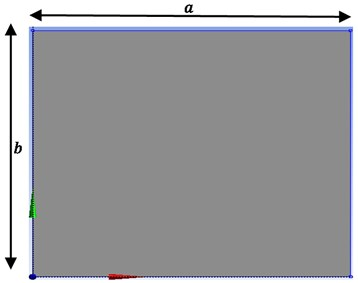Fig. 2. Acoustic enclosure and velocity boundary condition application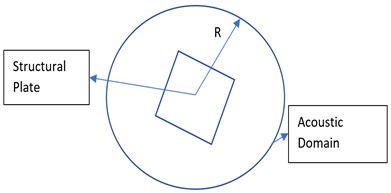#### 4. Results and discussion

The modal analysis is carried out to calculate the natural frequency and mode shape of the plate. In modal analysis 20 modes have been extracted but due to space constraints only first 6 modes have been shown in Fig. 3.

In the harmonic response analysis upon exciting of plate using concentrated force of 1 N, the normal nodal velocity response is calculated. The normal velocity response that is to be imported in harmonic acoustic analysis is shown in Fig. 4.

Fig. 3. First 6 mode shapes along with natural frequency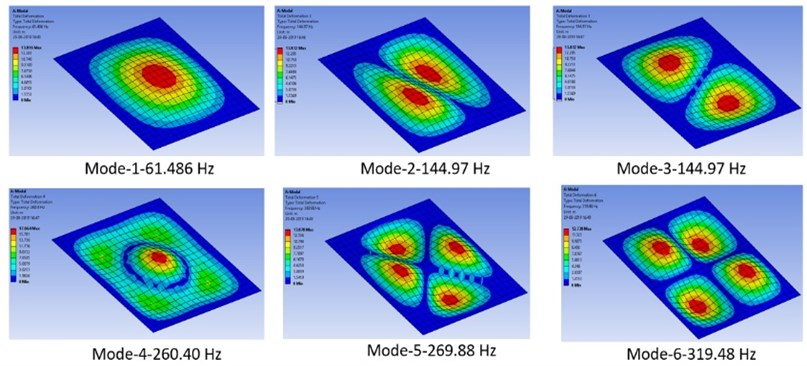Fig. 4. Frequency response of vibrating plate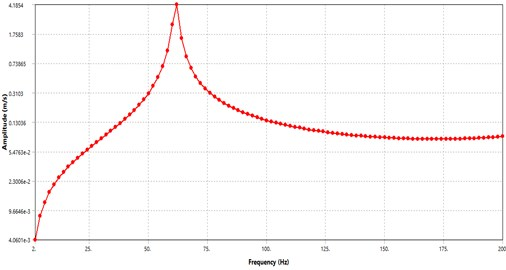#### 4.1.1. Sound pressure level

The sound pressure level is calculated using Rayleigh integral. Sound pressure level is a parameter that signifies the amount of sound radiated from vibrating structures. For lower frequency upto 20 Hz rate of increment in SPL is higher as compared to that of frequencies greater than 20 Hz.

Sound pressure level obtained at far-field location (0, 0, 0.8) m is shown in Fig. 5. It is found that sound power level ranges from 22.672 dB to 72.542 dB.

Fig. 5. Sound pressure level vs frequency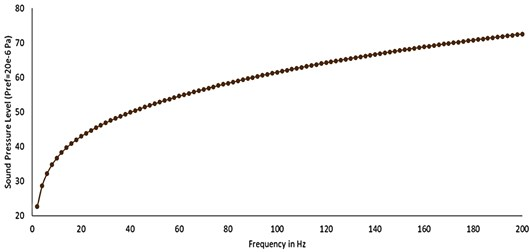#### 4.1.2. Sound power level

Sound power level is a critical sound radiation parameter that defines the radiation efficiency of any structure. Upon calculating the sound power level at (0, 0, 0.8), it is found that the sound power level ranges from 42.164 dB to 78.002 dB.

Fig. 6. Sound power level vs frequency plots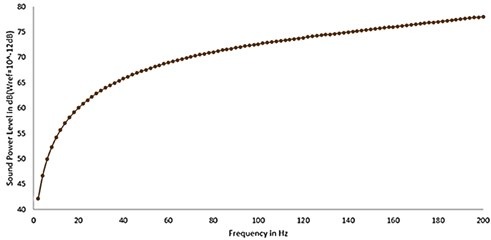Sound radiation efficiency is the factor that characterizes the efficiency of any sound radiation system. It is actually the ratio between sound power level calculated to the baffled sound power level calculated assuming the structure to be the piston. It is obvious from Fig. 7 that radiation efficiency is minimum at frequency 60.5 Hz.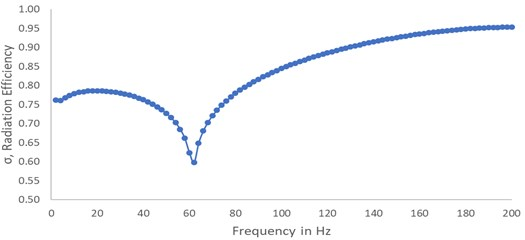#### 5. Conclusions

In this paper acoustic radiation of vibrating simply supported rectangular plate placed in an infinite baffle have been analyzed numerically using Ansys19.2. The vibration characteristics of the plate due to forcing function gives us the vibrating normal velocity at each nodes of the plate numerically. These nodal velocities are applied as boundary condition for harmonic acoustic analysis in Ansys19.2. The sound radiation parameter as calculated and reported are sound pressure level and sound power level and sound radiation efficiency and it is found that far field acoustic pressure level at point (0, 0, 0.8) is found to range between 22 dB to 72 dB while sound power level ranged from 42 dB to 78.002 dB for a frequency range of interest 0 to 200 Hz. The sound radiation parameters are calculated are suitable for noiseless environment as there below the critical sound power level.

1. Akay A., Latcha M. A theoretical analysis of transient sound radiation from a clamped circular plate. Journal of Applied Mechanics, Vol. 51, 1984, p. 41-47. [Publisher]
2. Cummings A. Sound radiation from a plate into a porous medium. Journal of Sound and Vibration, Vol. 247, Issue 3, 2001, p. 389-406. [Publisher]
3. Wallace C. E. Radiation resistance of a rectangular panel. The Journal of the Acoustical Society of America, Vol. 51, 1972, p. 946. [Publisher]
4. Fahy Frank, Gardonio Paolo Sound and Structural Vibration Radiation, Transmission and Response. 7th Edition, Academic Press, Southampton, England, 2001. [Search CrossRef]
5. Laulagnet B. Sound radiation by a simply supported unbaffled plate. The Journal of the Acoustical Society of America, Vol. 103, Issue 5, 1998, p. 2451-2462. [Publisher]
6. Kirkup Stephen Introduction to the Boundary Element Method; Boundary Element Solution of Laplace Problems: BEMLAP Fortran Package Introduction to the Boundary Element Method. Report SMK-18-001, 2018. [Search CrossRef]
7. Baron Rayleigh Strutt, William John The Theory of Sound. London, Vol. 1, 2015. [Search CrossRef]
8. ANSYSA® Academic Research Mechanical, Release 19.2, Help System, Acoustic Analysis Guide ANSYS, Inc. Ansys Inc., Southpointe 2600 ANSYS Drive Canonsburg, PA 15317 USA, 2018. [Search CrossRef]
9. Keltie R. F., Peng H. The effects of modal coupling on the acoustic power radiation from panels. Journal of Vibration, Acoustics, Stress, and Reliability in Design, Vol. 109, Issue 86, 1987, p. 48-54. [Publisher]
10. Mattei P. O. Sound radiation by baffled and constrained plates. Journal of Sound and Vibration, Vol. 179, 1995, p. 63-77. [Publisher]
11. Zaman I., Rozlan S. A. M., Yusoff A., Madlan M. A., Chan S. W. Theoretical modelling of sound radiation from plate. IOP Conference Series: Materials Science and Engineering, Malaysia, 2017. [Publisher]

#### Cited By

 Materials Today: Proceedings R. Kiran Kumar Reddy, Nivish George, Sreejith Mohan, Vinod Bhagat, M.P. Arunkumar 2022